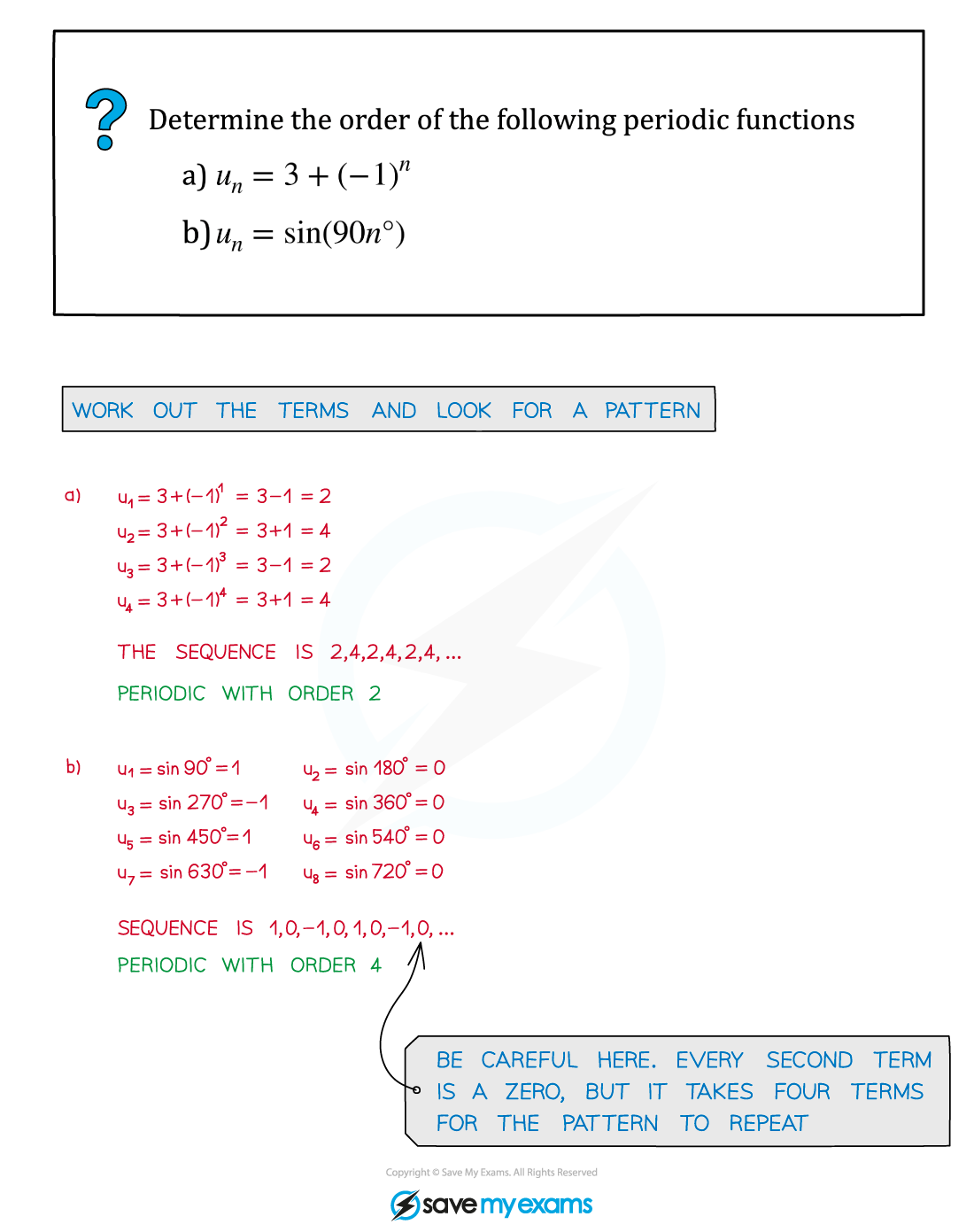# AQA A Level Maths: Pure复习笔记4.5.1 Language of Sequences & Series

### Language of Sequences & Series

#### What is a sequence?

• A sequence is an ordered set of numbers with a rule for finding all the numbers in the sequence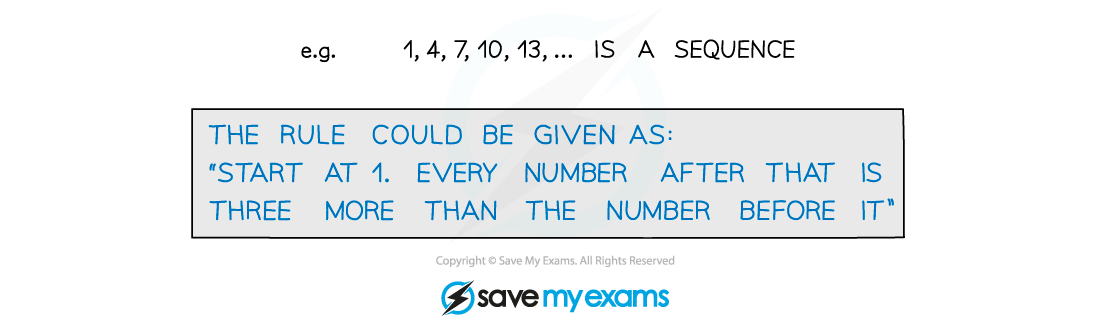• The numbers in a sequence are called terms
• The terms of a sequence are often referred to by letters with a subscript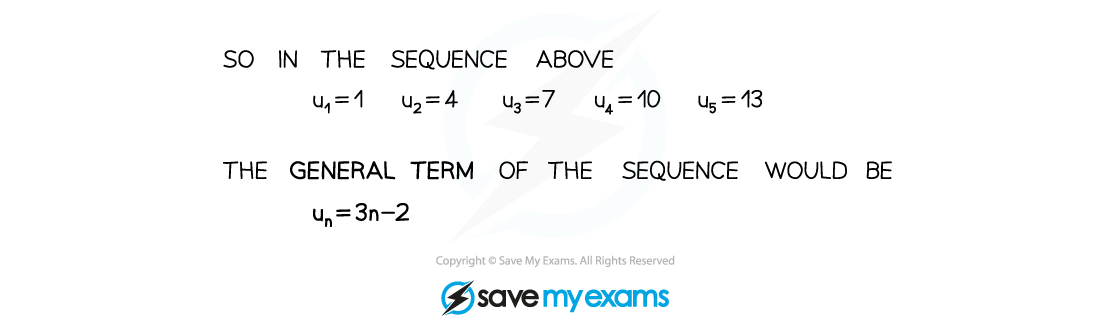#### What is a series?

• You get a series by summing up the terms in a sequence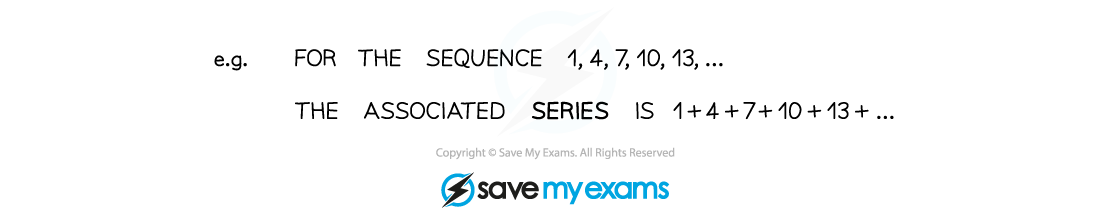• We use the notation Sn to refer to the sum of the first n terms in the seriesie.   Sn = u1 + u2 + u3 + … + un#### Increasing, decreasing and periodic sequences

• A sequence is increasing if un+1 > un for all positive integers n – ie if every term is greater than the term before it
• A sequence is decreasing if un+1 < un for all positive integers n – ie if every term is less than the term before it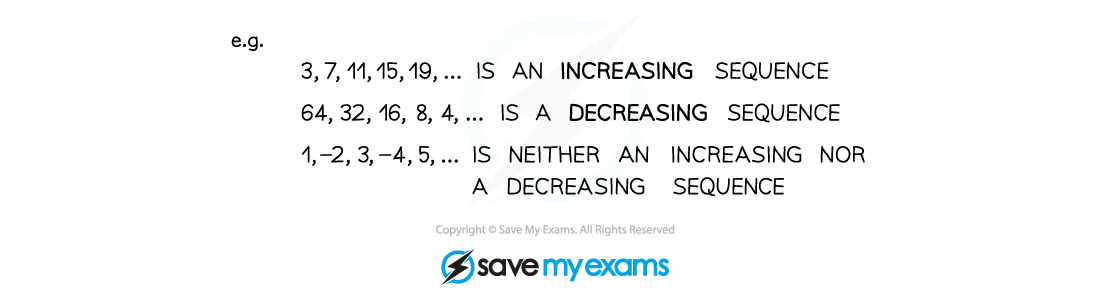• A sequence is periodic if the terms repeat in a cycle
• The order (or period) of a periodic sequence is the number of terms in each repeating cycle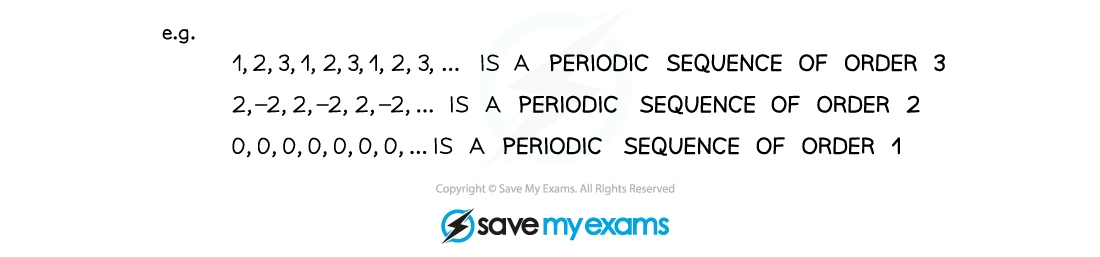#### Exam Tip

Look out for sequences defined by trigonometric functions – this can be a way of 'hiding' a periodic function.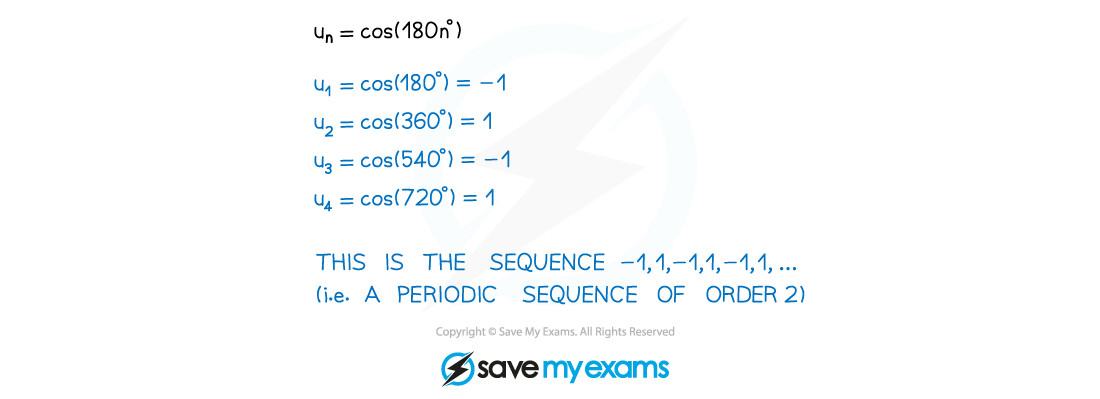#### Worked Example